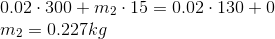# AP Physics C: Mechanics : Understanding Conservation of Momentum

## Example Questions

### Example Question #1 : Understanding Conservation Of Momentum

In an inelastic collision, which combination of quantities is conserved?

Mass, kinetic energy, and momentum

Mass and momentum

Momentum and kinetic energy

Mass and potential energy

Kinetic energy and potential energy

Mass and momentum

Explanation:

In a perfectly inelastic collision, the two colliding objects stick together; the two colliding objects deform, but mass is still conserved. Momentum is conserved during collisions of any sort, including inelastic collisions.

Kinetic energy is reduced during an inelastic collision, and is only conserved in elastic collisions. During inelastic collisions, some kinetic energy is lost to the environment in the form of heat or sound.

The problem does not give any information regarding position, and thus we cannot comment on any changes or lack of changes in potential energy.

### Example Question #1 : Momentum

In an elastic collision, what combination of quantities is conserved?

Momentum, kinetic energy, and potential energy

Mass and momentum

Potential energy and kinetic energy

Momentum, kinetic energy, and potential energy

Mass, momentum, and kinetic energy

Mass, momentum, and kinetic energy

Explanation:

The primary difference between elastic and inelastic collisions is the conservation of kinetic energy. Kinetic energy is conserved in elastic collisions, but is not conserved in inelastic collisions. Momentum is always conserved, regardless of collision type. Mass is conserved regardless of collision type as well, but the mass may be deformed by an inelastic collision, resulting in the two original masses being stuck together.

There is no description in the problem reagrding position, so we cannot comment on potential energy.

### Example Question #3 : Understanding Conservation Of Momentum

A 200kg car is traveling west at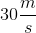. It collides with a 150kg car that was at rest. Following the collision, the second car moves with a velocity of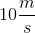west. Assuming that the collision is elastic, what is the velocity of the first car after the collision?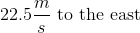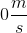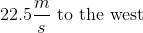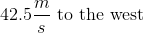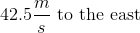Explanation:

The collision is assumed to be elastic, so both momentum and kinetic energy are conserved. Use the law of conservation of momentum: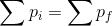Momentum is the product of velocity and mass: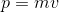We can expand the summation for the initial and final conditions: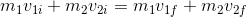Use the given values to fill in the equation and solve for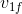: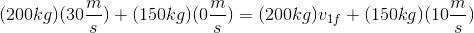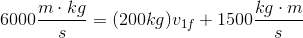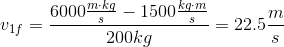Since the final velocity is positive, we know that the car is still traveling toward the west.

### Example Question #4 : Understanding Conservation Of Momentum

Two train cars, each with a mass of 2400 kg, are traveling along the same track. One car is traveling with a velocity of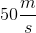east, while the other travels with a velocity of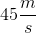west. The two cars collide and stick together as one mass. What is the magnitude and direction of the resulting velocity?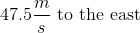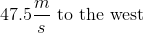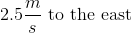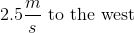Explanation:

Use the law of conservation of momentum:Momentum is the product of velocity and mass:We can expand the summation for the initial and final conditions:Note that we are working with an inelastic collision, meaning that the two masses stick together after the collision. Because of this, they will have the same final velocity: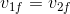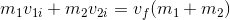Use the given values to fill in the equation and solve for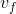. Keep in mind that we must designate a positive direction and a negative direction. We will use east as positive and west as negative.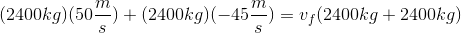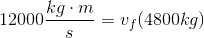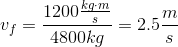Since the final velocity is positive, we can determine that they train cars are traveling toward the east.

### Example Question #5 : Understanding Conservation Of Momentum

A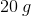bullet is fired at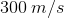at a block of wood that is moving in the opposite direction at a speed of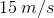. The bullet passes through the block and emerges with the speed of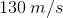, while the block ends up at rest.

What is the mass of the block?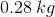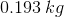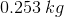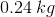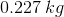Explanation:

This problem is a conservation of momentum problem. When doing these types of problems, the equation to jump to is:It is given to us that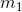isor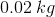,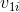is,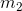is unknown,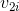is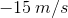.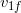isand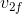is.

With all this information given, the only unknown is.

Plugging everything in, we get: